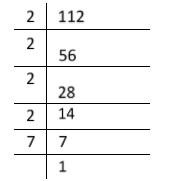QuestionAnswers

# Explain why $15 \times 7 + 7$ is a composite number.Verified
128.4k+ views
Hint: A composite number is a positive integer that can be formed by multiplying two smaller positive integers. That integers has at least one divisor other than $1$ and itself.

Complete step by step solution::
The given number is: $15 \times 7 + 7$
There are two terms in the given number and $7$is a common factor.
We take $7$as common, then we have
$15 \times 7 + 7 = 7\left( {15 \times 1 + 1} \right)$
$15 \times 7 + 7 = 7\left( {15 + 1} \right)$
$15 \times 7 + 7 = 7\left( {16} \right)$
$15 \times 7 + 7 = 112$
When we factorize$112$, we will get$112 = 2 \times 2 \times 2 \times 2 \times 7$
$112 = {2^4} \times 7$
This number is divisible by $2,4,7,8$and $16$, which means that it has more than two factors.
Therefore, $15 \times 7 + 7$ is a composite number
Additional information: the divisibility rule is given below:
(i) Divisibility rule of $2$: Which states that for a number to be divisible by $2$, the unit digit must have $0,2,4,6$or $8$ in units place.
(ii) Divisibility rule of $4$: Which states that for a number to be divisible by $4$, the unit and tens digit should be divisible by $4$
(iii) Divisibility rule of $7$: We need to double the last digit of the number and then subtract it from the remaining number. If the result is divisible by $7$, then the original number will also be divisible by $7$.

Note: Different types of numbers are:
(i) Natural number: $1,2,3,4,$------
(ii) Whole number:$\;0,1,2,3,4,$ ------
(iii) Integers: $- 4, - 3, - 2, - 1,0,1,2,3,4,$-----
(iv) Positive integers: $1,2,3,$-----
(v) Negative integers: $- 4, - 3, - 2, - 1$